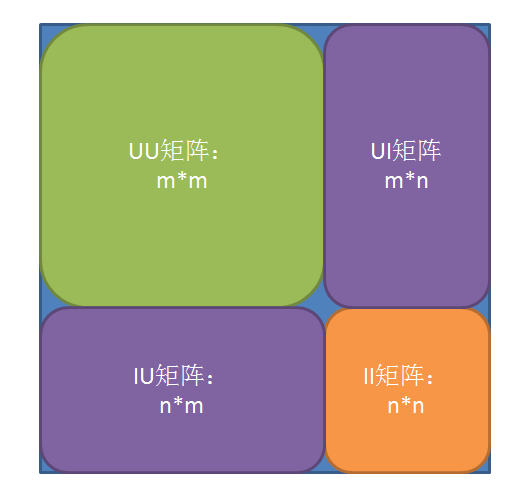推荐系统学习之概率算法及其增量算法

0.絮

1. 前些阵子了参加了一个天池的推荐比赛，做了一些，想了很多，因此有了下文。顺便了解过一点markdown，发现还挺好的。这次用用看。

2.环境设定

PR(PageRank)中，只有一个要素：网页，一种关系：网页与网页之间的Arrow(矢量：既有大小又有方向)，而在推荐中涉及两个要素：U 用户I 物品，一种关系：用户作用（购买、浏览、评价）了物品的Arrow。为了能够尽情的抄袭 PR我们做以下两点假设：
- UI的地位是等价，我们可以尽情的把它们当做Page来处理。
- 每一个Array（作用）都是双向的 。

2.1.转移矩阵T

PR类似，转移矩阵T是这个方法中的关键部分。转移矩阵如下图所示：分为四部分。• UU矩阵：用户到用户之间的转移矩阵，为0矩阵，m为用户个数。
• UI矩阵 ：用户到物品的转移矩阵，用户购买的物品取值为1/NumI(NumI为用户购买物品数量)。
• IU矩阵 ：物品到用户的转移矩阵，购买过该物品的用户位置取值1/NumU(NumU为该物品的购买用户数)。
• II矩阵 ：商品到商品的转移矩阵，为0矩阵，n为物品个数。
整体是一个（m+n）的方阵，前面m个是用户的部分，后面n个为商品的部分。

2.2得分矩阵 S

T矩阵类似也分为4个部分：

• UU矩阵：向用户到用户的推荐得分。
• UI矩阵 ：向用户推荐物品的得分矩阵。
• IU矩阵 ：向物品推荐用户的得分矩阵。
• II矩阵 ：向商品推荐商品的得分矩阵。
• 整体是一个（m+n）的方阵，初始得分记做S0，为单位矩阵

1. Sn+1=SnT$S_{n+1} = S_nT$
2. Sn=S0Tn$S_n=S_0 T^n$

1. 当n为偶数时，Sn中的紫色块为0矩阵，可以解释为同类元素之间的相似性（包括物品相似性和用户相似性）。
2. 当n为奇数时，Sn中的非紫色块为0矩阵，可以解释为不同类元素之间的相似性（向用户推荐物品得分以及向物品推荐用户得分）。

3.该方法的简单性质

3.1Sn 可计算

Sn=S0Tn$S_n=S_0 T^n$敬爱的数学老师曾经告诉我们一个技巧：T=PAP1$T=PAP^{-1}$而这一过程称为矩阵对角化，如果使用矩阵求逆的方法来求A、P矩阵，其算法复杂度主要取决与这个求逆运算，而矩阵求逆运算的复杂度为O(n3)$O(n^3)$(考虑到Coppermith_Winograd算法对矩阵乘法的简化，其复杂度应该可以降低到O(n2.37)$O(n^{2.37})$左右)。而Sn=S0Tn=S0PAnP1$S_n=S_0 T^n=S_0PA^nP^{-1}$。其中A$A$为对角矩阵，其An$A^n$容易计算，复杂度为O(n)$O(n)$。因此最终复杂度由矩阵的求逆运算决定。

3.2增量ΔSn$\Delta S_n$可描述

• ΔS3=S0ΔT+T03S0T3$\Delta S_3=S_0 （\Delta T+T_0）^3-S_0 T^3$
S0ΔTTT+TΔTT+TTΔT$\approx S_0 （\Delta TTT+T\Delta TT+TT\Delta T）$（当ΔT$\Delta T$较小时，忽略ΔT$\Delta T$的高阶项）

ΔS3$\Delta S_3$ΔSn$\Delta S_n$ 推广的体力活我就省略了，诸位记住矩阵对角化可以简化计算就行了，下面简要讨论计算增量。

1. T矩阵对应用户行的N1个非空元素，值由1/N1变为1/N2,变化量为N2N1N2N1$\frac {N2-N1}{N2N1}$
2. T矩阵对应用户行增加N2-N1个非零元素，值为1/N2。

5.小结

1. 正向思维方法：先发现（假设）事物符合某个理论（假设的不好就是谬论）规律从而得到H$H$函数，然后根据X的值得到Y=H(X)$Y=H(X)$的对应输出结果。
2. 逆向思维方法：先有很大的训练集合（也就是X和Y），然后不停的修改函数H，得到一个合适的H，然后根据X的值得到Y=H(X)$Y=H(X)$的对应输出结果。

We read the world wrong and say that it deceives us. 理性参阅